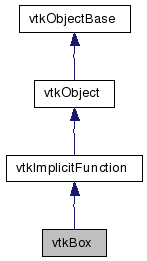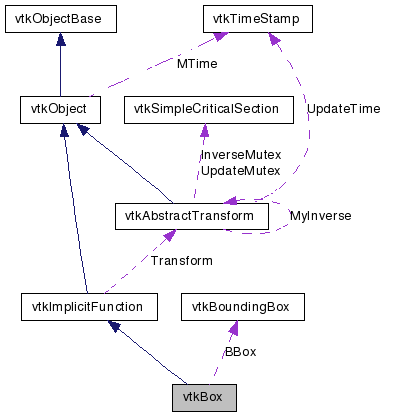# vtkBox Class Reference

`#include <vtkBox.h>`

Inheritance diagram for vtkBox:[legend]
Collaboration diagram for vtkBox:[legend]

## Detailed Description

implicit function for a bounding box

vtkBox computes the implicit function and/or gradient for a axis-aligned bounding box. (The superclasses transform can be used to modify this orientation.) Each side of the box is orthogonal to all other sides meeting along shared edges and all faces are orthogonal to the x-y-z coordinate axes. (If you wish to orient this box differently, recall that the superclass vtkImplicitFunction supports a transformation matrix.) vtkCube is a concrete implementation of vtkImplicitFunction.

vtkCubeSource vtkImplicitFunction
Tests:
vtkBox (Tests)

Definition at line 39 of file vtkBox.h.

## Public Types

typedef vtkImplicitFunction Superclass

## Public Member Functions

virtual const char * GetClassName ()
virtual int IsA (const char *type)
void PrintSelf (ostream &os, vtkIndent indent)
void EvaluateGradient (double x, double n)
void SetXMax (double p)
void SetXMax (double x, double y, double z)
void GetXMax (double p)
void GetXMax (double &x, double &y, double &z)
void SetBounds (double xMin, double xMax, double yMin, double yMax, double zMin, double zMax)
void SetBounds (double bounds)
void GetBounds (double &xMin, double &xMax, double &yMin, double &yMax, double &zMin, double &zMax)
void GetBounds (double bounds)
double * GetBounds ()
void AddBounds (double bounds)
double EvaluateFunction (double x)
double EvaluateFunction (double x, double y, double z)
void SetXMin (double p)
void SetXMin (double x, double y, double z)
void GetXMin (double p)
void GetXMin (double &x, double &y, double &z)

## Static Public Member Functions

static int IsTypeOf (const char *type)
static vtkBoxSafeDownCast (vtkObject *o)
static vtkBoxNew ()
static char IntersectBox (double bounds, double origin, double dir, double coord, double &t)

vtkBox ()
~vtkBox ()

## Protected Attributes

vtkBoundingBoxBBox
double Bounds 

## Member Typedef Documentation

Reimplemented from vtkImplicitFunction.

Definition at line 42 of file vtkBox.h.

## Constructor & Destructor Documentation

 vtkBox::vtkBox ( ) ` [protected]`

 vtkBox::~vtkBox ( ) ` [protected]`

## Member Function Documentation

 virtual const char* vtkBox::GetClassName ( ) ` [virtual]`

Reimplemented from vtkImplicitFunction.

 static int vtkBox::IsTypeOf ( const char * name ) ` [static]`

Return 1 if this class type is the same type of (or a subclass of) the named class. Returns 0 otherwise. This method works in combination with vtkTypeRevisionMacro found in vtkSetGet.h.

Reimplemented from vtkImplicitFunction.

 virtual int vtkBox::IsA ( const char * name ) ` [virtual]`

Return 1 if this class is the same type of (or a subclass of) the named class. Returns 0 otherwise. This method works in combination with vtkTypeRevisionMacro found in vtkSetGet.h.

Reimplemented from vtkImplicitFunction.

 static vtkBox* vtkBox::SafeDownCast ( vtkObject * o ) ` [static]`

Reimplemented from vtkImplicitFunction.

 void vtkBox::PrintSelf ( ostream & os, vtkIndent indent ) ` [virtual]`

Methods invoked by print to print information about the object including superclasses. Typically not called by the user (use Print() instead) but used in the hierarchical print process to combine the output of several classes.

Reimplemented from vtkImplicitFunction.

 static vtkBox* vtkBox::New ( ) ` [static]`

Construct box with center at (0,0,0) and each side of length 1.0.

Reimplemented from vtkObject.

 double vtkBox::EvaluateFunction ( double x ) ` [virtual]`

Evaluate box defined by the two points (pMin,pMax).

Implements vtkImplicitFunction.

 double vtkBox::EvaluateFunction ( double x, double y, double z ) ` [inline]`

Evaluate box defined by the two points (pMin,pMax).

Reimplemented from vtkImplicitFunction.

Definition at line 51 of file vtkBox.h.

 void vtkBox::EvaluateGradient ( double x, double n ) ` [virtual]`

Evaluate the gradient of the box.

Implements vtkImplicitFunction.

 void vtkBox::SetXMin ( double p ) ` [inline]`

Set / get the bounding box using various methods.

Definition at line 113 of file vtkBox.h.

 void vtkBox::SetXMin ( double x, double y, double z )

Set / get the bounding box using various methods.

 void vtkBox::GetXMin ( double p )

Set / get the bounding box using various methods.

 void vtkBox::GetXMin ( double & x, double & y, double & z )

Set / get the bounding box using various methods.

 void vtkBox::SetXMax ( double p ) ` [inline]`

Definition at line 118 of file vtkBox.h.

 void vtkBox::SetXMax ( double x, double y, double z )

 void vtkBox::GetXMax ( double p )

 void vtkBox::GetXMax ( double & x, double & y, double & z )

 void vtkBox::SetBounds ( double xMin, double xMax, double yMin, double yMax, double zMin, double zMax )

 void vtkBox::SetBounds ( double bounds )

 void vtkBox::GetBounds ( double & xMin, double & xMax, double & yMin, double & yMax, double & zMin, double & zMax )

 void vtkBox::GetBounds ( double bounds )

 double* vtkBox::GetBounds ( )

 void vtkBox::AddBounds ( double bounds )

A special method that allows union set operation on bounding boxes. Start with a SetBounds(). Subsequent AddBounds() methods are union set operations on the original bounds. Retrieve the final bounds with a GetBounds() method.

 static char vtkBox::IntersectBox ( double bounds, double origin, double dir, double coord, double & t ) ` [static]`

Bounding box intersection modified from Graphics Gems Vol I. The method returns a non-zero value if the bounding box is hit. Origin starts the ray, dir is the vector components of the ray in the x-y-z directions, coord is the location of hit, and t is the parametric coordinate along line. (Notes: the intersection ray dir is NOT normalized. Valid intersections will only occur between 0<=t<=1.)

## Member Data Documentation

 vtkBoundingBox* vtkBox::BBox` [protected]`

Definition at line 103 of file vtkBox.h.

 double vtkBox::Bounds` [protected]`

Definition at line 104 of file vtkBox.h.

The documentation for this class was generated from the following file:

Generated on Sat Dec 27 13:17:54 2008 for VTK by1.5.6